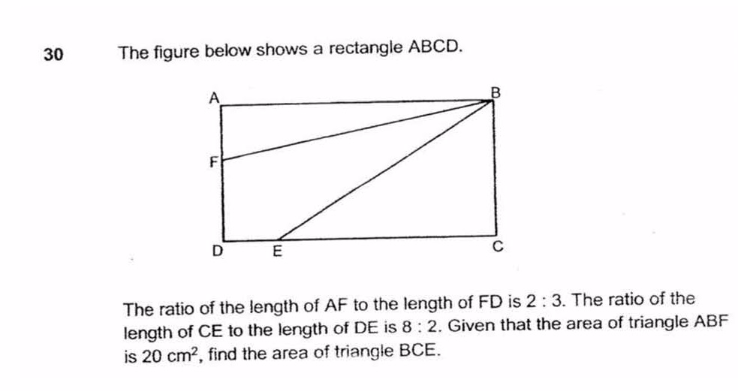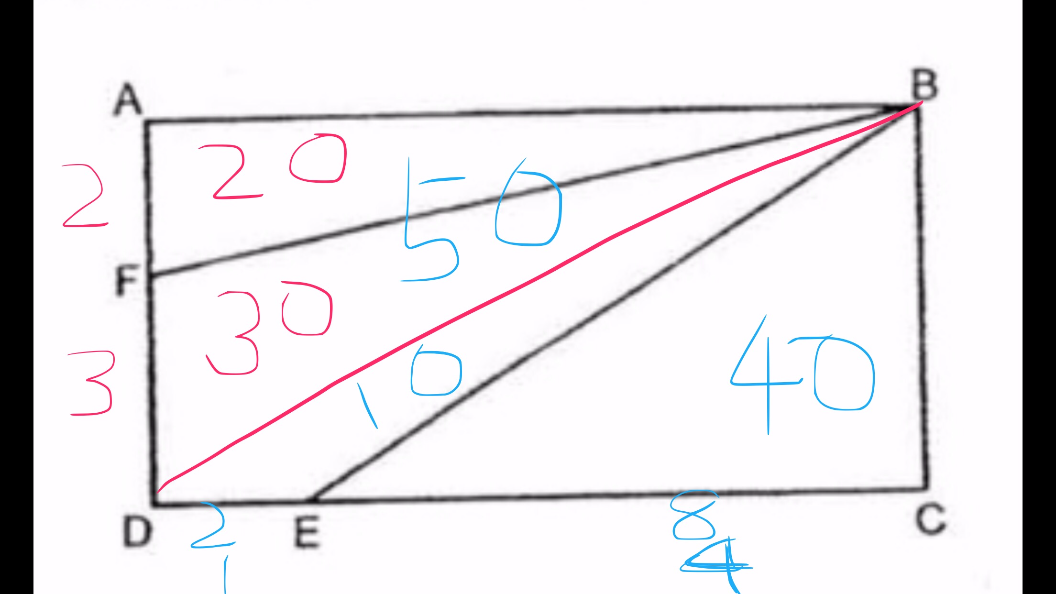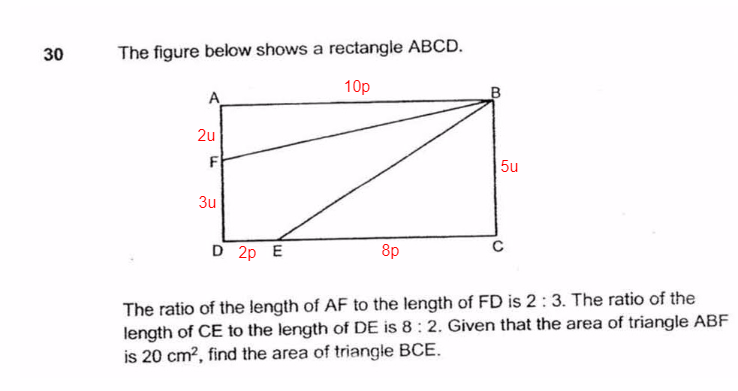# QuestionPlease help with this question.  Thank you.

3 Answers

# Answer1 Reply 0 Likes ✔Accepted Answer

It’s a clear solutions, thank you all.  Greatly appreciate it.

0 Replies 0 Likes

By drawing a line from point B to point D, the rectangle is divided into 2 halves.
area of ABF = 2/5 x 1/2 x area of rectangle ABCD (The ratio of the length of AF to the length of FD is 2 : 3.)
2/10 = 1/5
1/5 x area of rectangle ABCD ——- 20
area BCE = 8/10 = 4/5 x 1/2 x  area of the rectangle ABCD (The ratio of the length of CE to the length of DE is 8 : 2)
4/10 = 2/5
2/5 x area of rectangle ABCD ——- 2 x 20 = 40

Ans : 40 sq cm.

0 Replies 1 LikeArea ΔABF = ½ × 2u × 10p = 10up

10up = 20 cm²

Area ΔBCE = ½ × 5u × 8p = 20up

20up = 2 × 10up = 2 × 20 = 40 cm²

0 Replies 1 Like